# Head Swimming With Calculating Fractions

If your profession requires you preform lots of operations with fractions then these apps are what you need to be efficient and speedy. Whatever your fraction calculating needs we got you covered.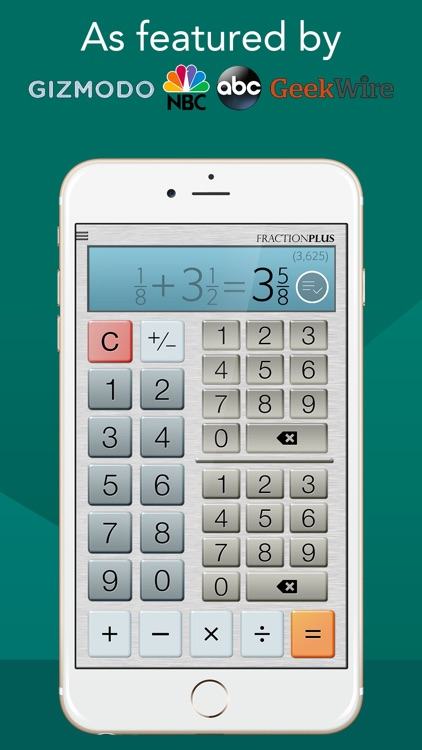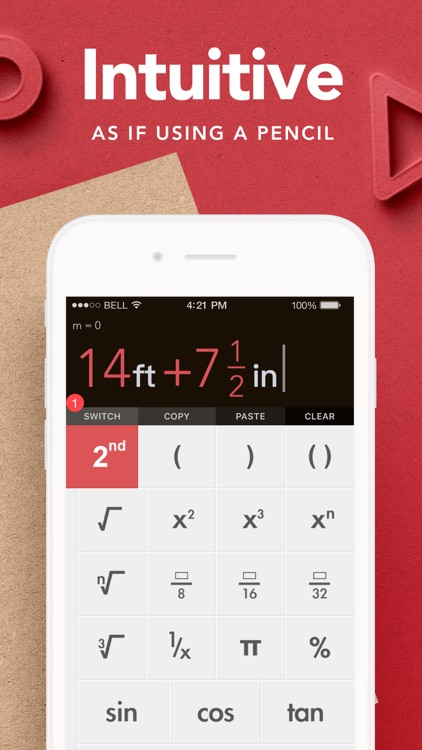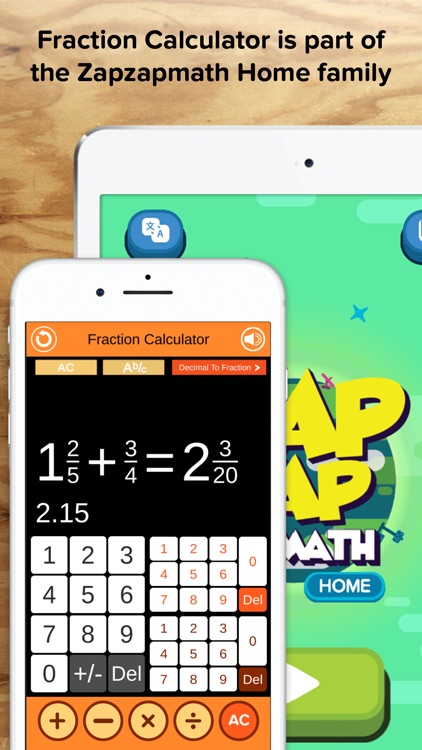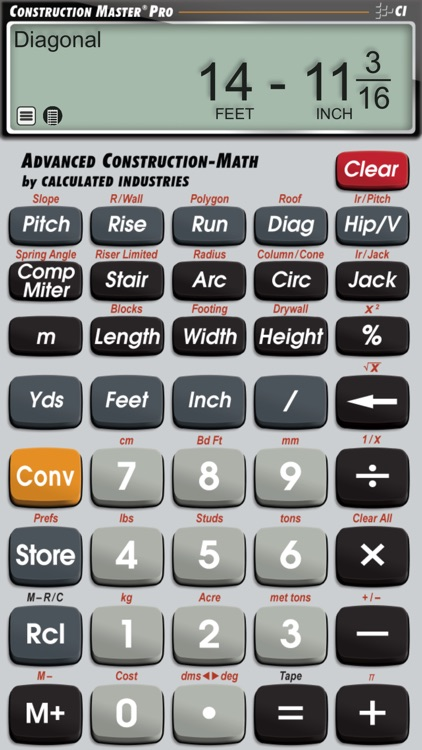## Best Apps

Highest scored apps in the category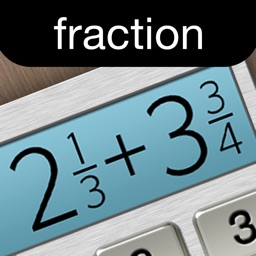### Fraction Calculator Plus

DigitAlchemy LLC

I'm Fraction Calculator Plus and I'm the best and easiest way to deal with everyday fraction problems

I'm Fraction Calculator Plus and I'm the best and easiest way to deal with everyday fraction problems. Whether you're checking fractions homework, preparing recipes, or working on craft or even construction projects, I can help:

- Wish you could find the time to check your kids' math homework? Now you can - checking fraction math takes just seconds!
- Need...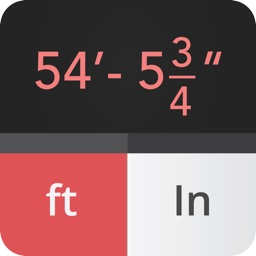### Fraction Calculator

xNeat.com

Award winning Feet Inch Fraction Calculator & Metric converter for iPhone and iPad

Award winning Feet Inch Fraction Calculator & Metric converter for iPhone and iPad. Designed with simplicity, usability and beauty in mind!

FEATURES:

- Elegant & Intuitive interface
- Decimal to fraction converter
- Fraction to decimal conversion
- Displays both the equation & the result at the same time
- Advanced editing by easily going back and forth
- Can be added to Today Screen

Ideal for those...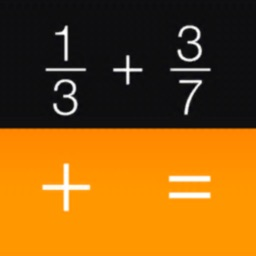### Fraction Calculator + Decimals

Visual Math Interactive Sdn. Bhd.

Introducing the world’s first Fraction Calculator packed with additional functions like reducing or simplifying fractions, fractions-to-decimals and a decimals-to-fractions calculator

Introducing the world’s first Fraction Calculator packed with additional functions like reducing or simplifying fractions, fractions-to-decimals and a decimals-to-fractions calculator. All these in one nifty app. Discover an easy way to solve everyday fraction problems. Add, subtract, multiply, divide and even convert fractions, quickly and clearly. Visual Math Interactive’s Fraction Calculator is an excellent homework help and business reference tool, with...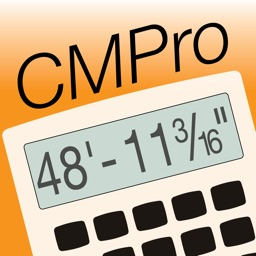### Construction Master Pro

Calculated Industries

Two in one: the official Construction Master® Pro from Calculated Industries for your iPhone and iPad includes two models: #4065 and #4080 (CM Pro with Trig)

Two in one: the official Construction Master® Pro from Calculated Industries for your iPhone and iPad includes two models: #4065 and #4080 (CM Pro with Trig). And while perhaps obvious, if you change from an iOS device to a non-iOS device, you WILL need to buy a new license as they are incompatible.

Advanced Feet-Inch-Fraction Construction-Math Calculator by Calculated Industries, built...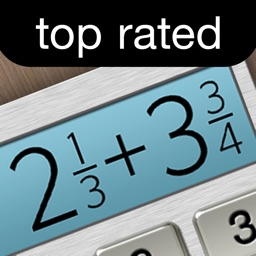### Fraction Calculator Plus #1

DigitAlchemy LLC

Fraction Calculator Plus is the world's favorite homework helper and the first of its kind, but does so much more

Fraction Calculator Plus is the world's favorite homework helper and the first of its kind, but does so much more. The perfect project companion, whether you're checking your math, preparing recipes in the kitchen, working on crafts or even construction projects, Fraction Calculator Plus is exactly what you're looking for.The genius of Fraction Calculator's original innovative design is in the...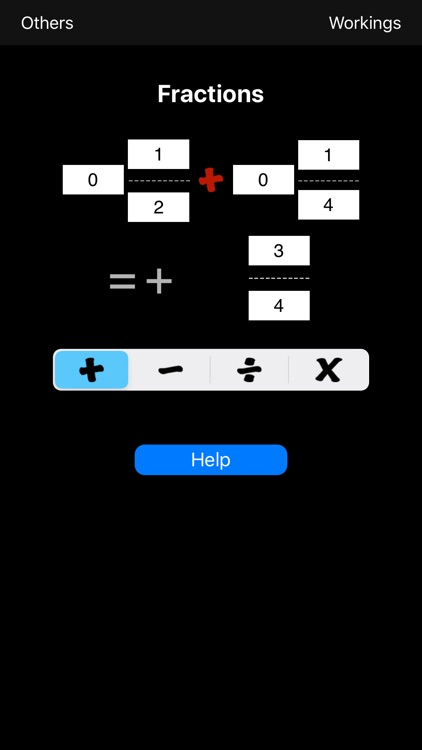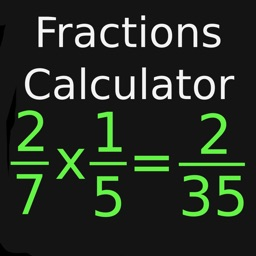### Fractions Calculator

Essence Computing

Do you struggle to work out fractions

Do you struggle to work out fractions? Do you need help in understanding how to add, subtract, divide and multiply fractions? Well if you do then this App is for you. Not only does it calculate the answers for you it also comprehensively explains how the answer was arrived at. Along the way the App explains about the Least Common...

## Also Good

Apps with average score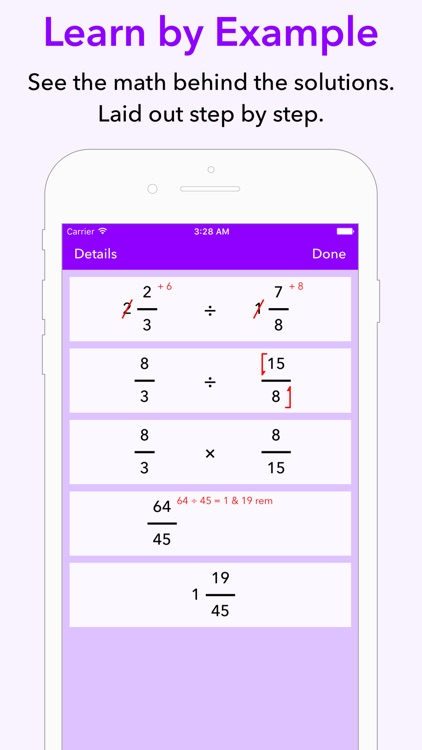### Fraction Help

Makes solving & learning fractions easy!

Fraction Help is a fraction calculator & educational tool that enables you to quickly solve fraction problems.

Features Include:

- Accurately solve fraction equations (addition, subtraction, multiplication & division)
- View detailed working, to learn how the final answered was determined*
- Work with mixed/partial...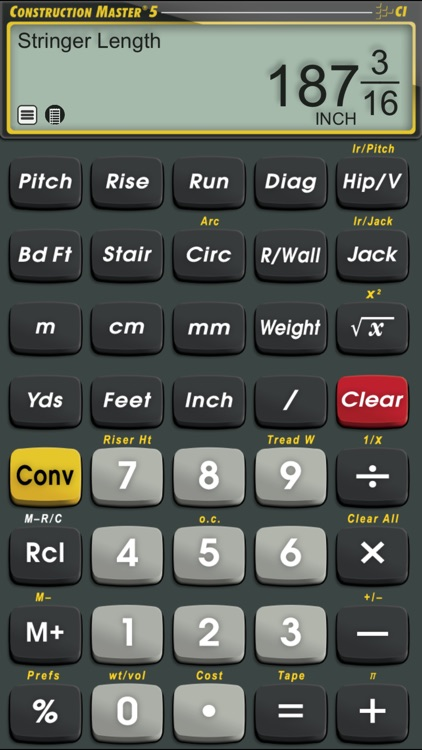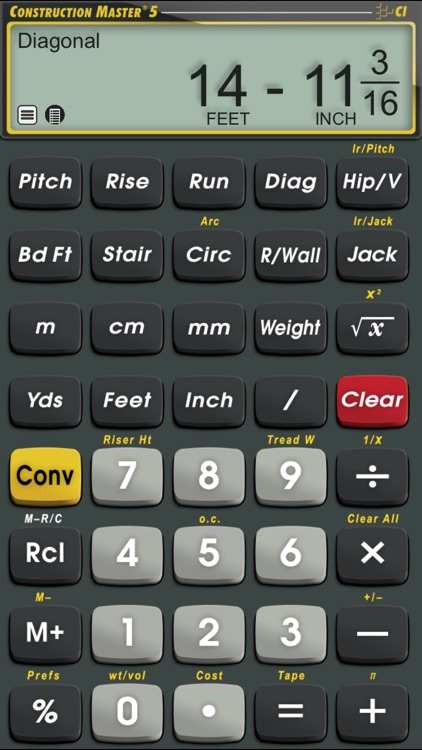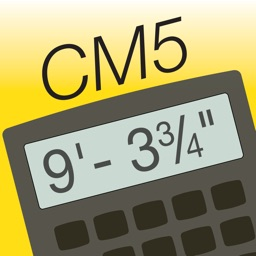### Construction Master 5

Calculated Industries

Feet-Inch-Fraction Construction-Math Calculator by Calculated Industries

The official Construction Master® 5 from Calculated Industries is now available for your iPhone and iPad. And while perhaps obvious, if you change from an iOS device to a non-iOS device, you WILL need to buy a new license as they are incompatible.

Built with the...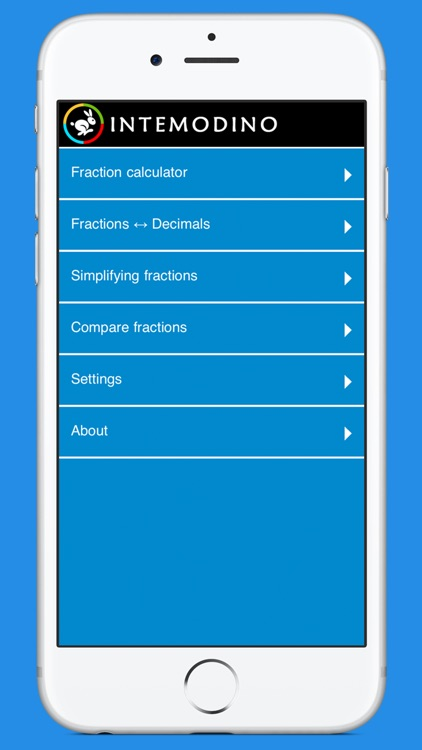### Fraction Calculator 4in1

Intemodino Group s.r.o.

Everything you need to convert, calculate, simplify and compare fractions in one app!

Fraction Converter and Calculator is perfect for engineers, carpenters, builders, students and anyone who needs a comprehensive fraction app.

► FRACTION CONVERTER
- Converts fractions to decimals and vice versa.
- Supports proper and improper fractions, mixed numbers, terminating and repeating...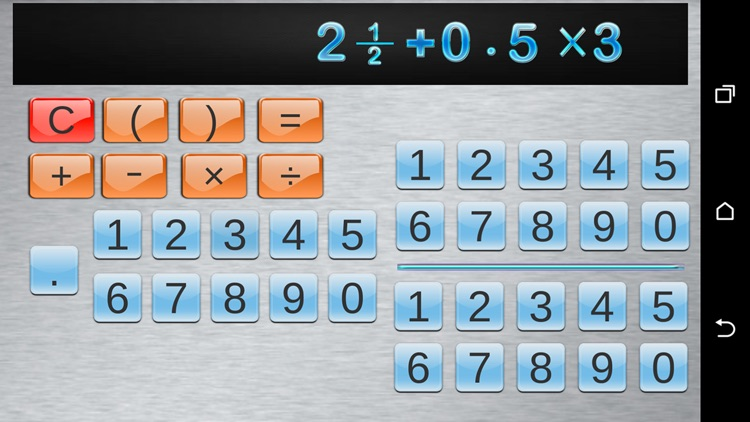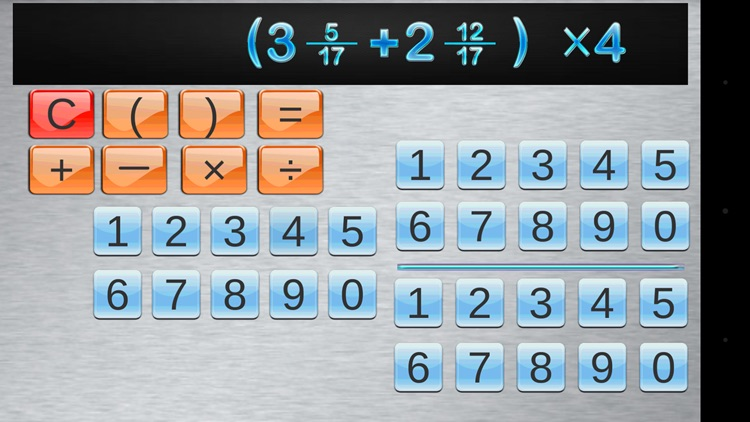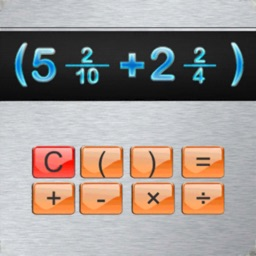### Fraction Calculator & progress

Chien Ming Chen

Easy to use fraction calculator.With the calculation process

The fraction calculator can used to check the student's homework.
Formula for reference only, because of the different regions have different algorithms.
Totally free.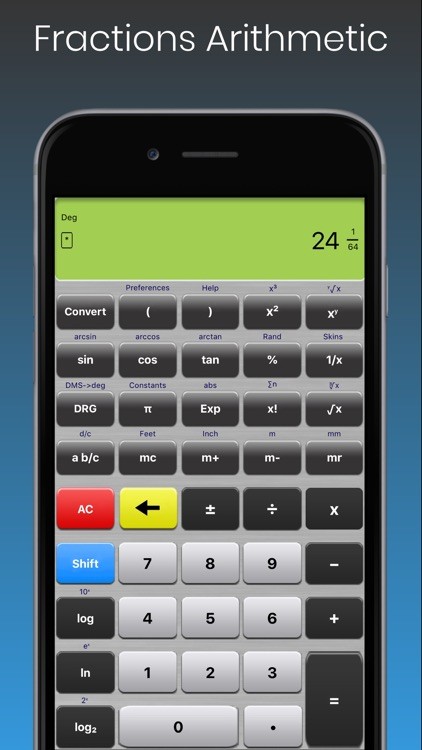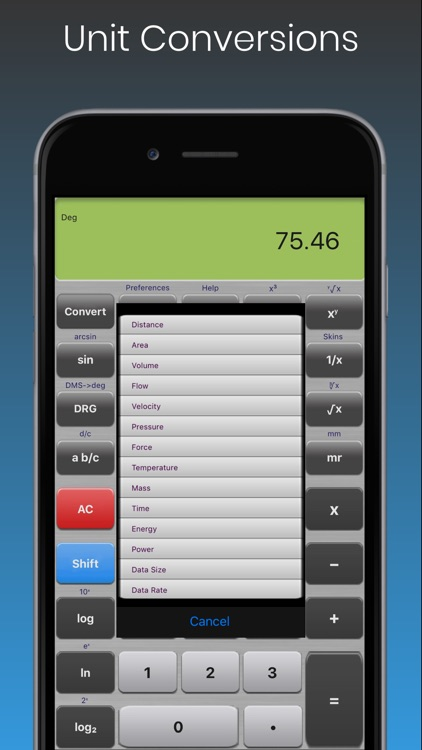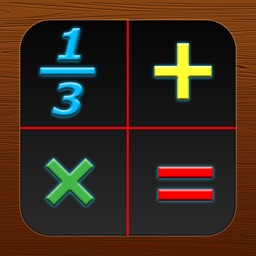### Scientific Calculator Elite

Cyberprodigy LLC

Scientific Calculator Elite™ is one of the most complete scientific calculators available for iOS. This fully featured scientific calculator looks and operates like the real thing.

Looking for fractions? Unit conversion and calculations with dimensional units? NO Ads? All this and more can be found with Scientific Calculator Elite™.

Scientific Calc...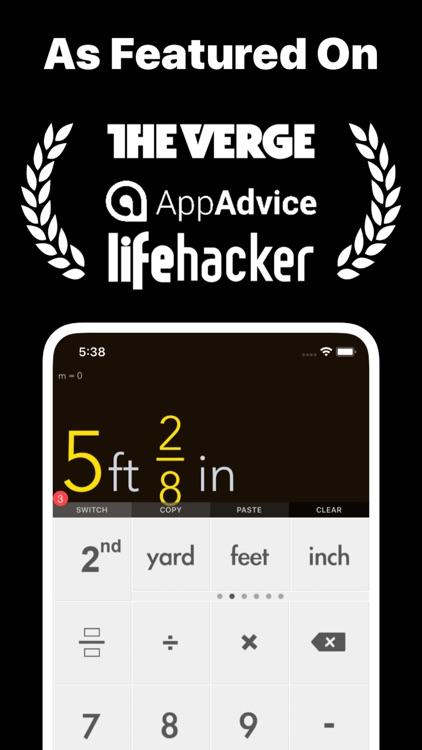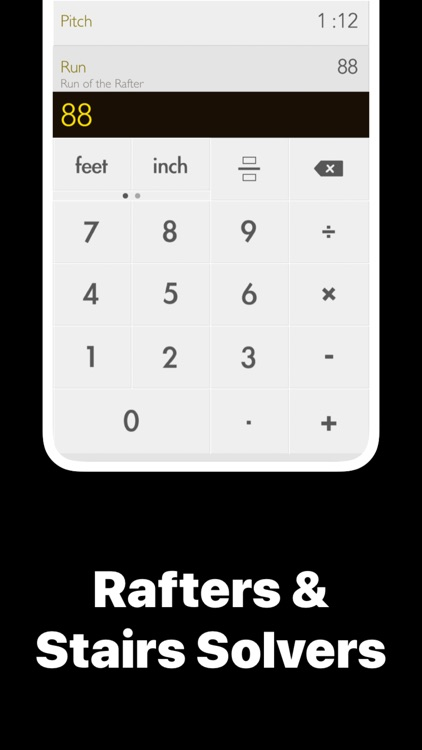### Construction Calculator

xNeat.com

Award Winning Feet Inch Fraction Construction Calculator for iPhone and iPad. Designed with simplicity, usability and beauty in mind!

FEATURES:

- Elegant & Intuitive interface
- Decimal to fraction converter
- Fraction to decimal conversion
- Displays both the equation & the result at the same time
- Advanced editing by easily going back and forth
-...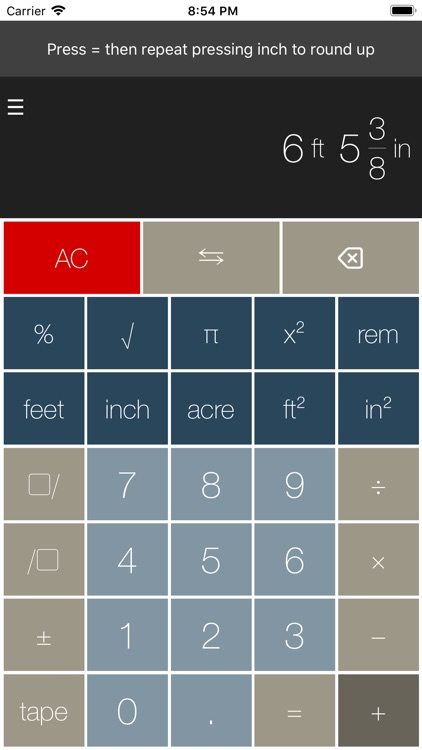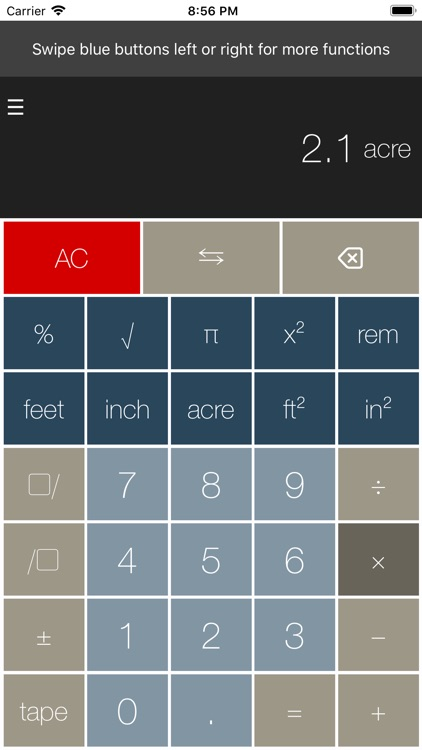### Feet&Inches Calculator

Megabyte Software Inc.

• Convert measurements in inches, feet, yards, meters, miles, kilometers, acres, square feet, hectare, cubic feet, board feet and more.
• Enter and calculate feet-inches and inches as fractions
• Option to round inches to the nearest 1/32nd, 1/16th, 1/8th, etc.
• Add and subtract measurements in different units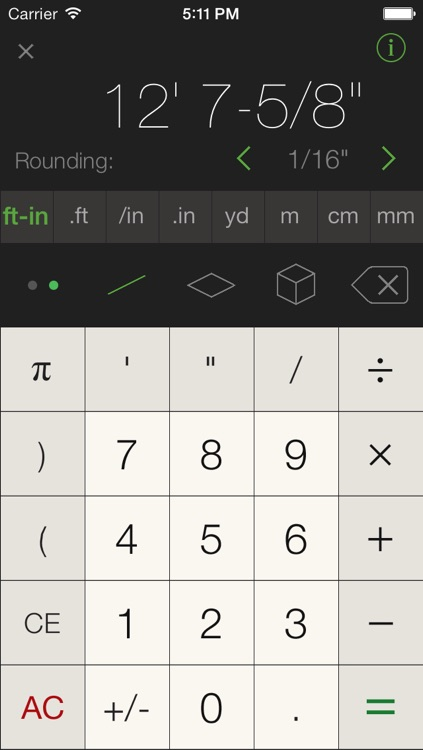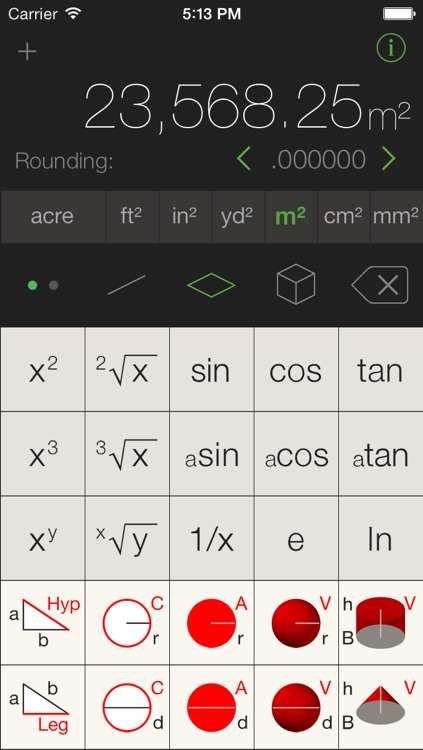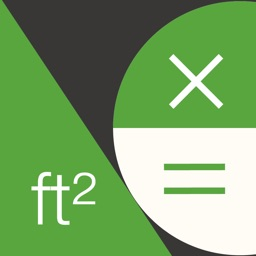### ArchiCalc

Thomas Boeman

ArchiCalc is a dimensional calculator for architects and builders. It is designed to make working with imperial and metric dimensions fluid and easy. Designed by an architect for architects, ArchiCalc works in a natural intuitive way with numbers, lengths, areas and volumes. Metric and Imperial units can be added together...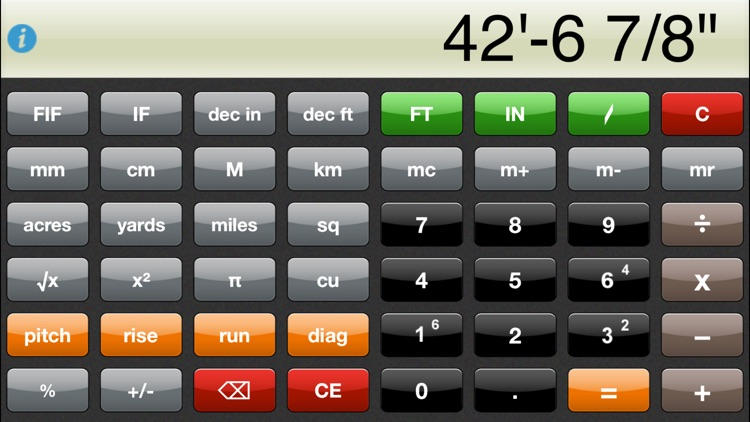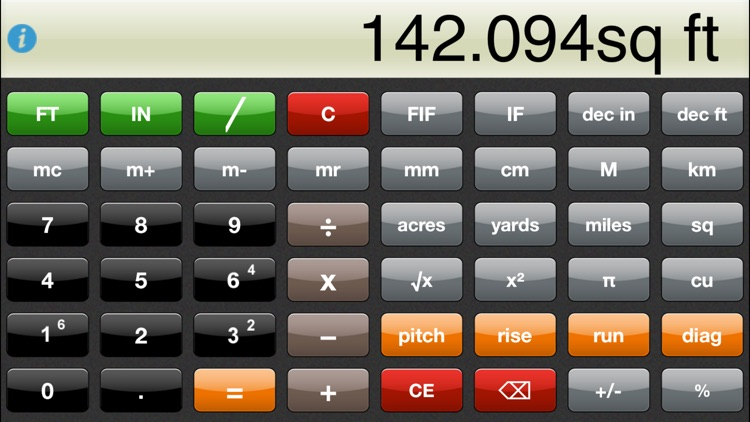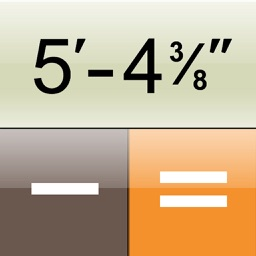### inchCALC+

River Studio

PRO LEVEL [FOOT • INCH • FRACTION] CALCULATOR

MACWORLD **** "inchCALC+'s ease of use coupled with its advanced features make this an uncommonly good addition to a crowded genre." 3/9/09

inchCALC+ is a State-of-the-Art Construction Calculator / Conversion Calculator for people who work with the FEET• INCH• FRACTION dimensions used on...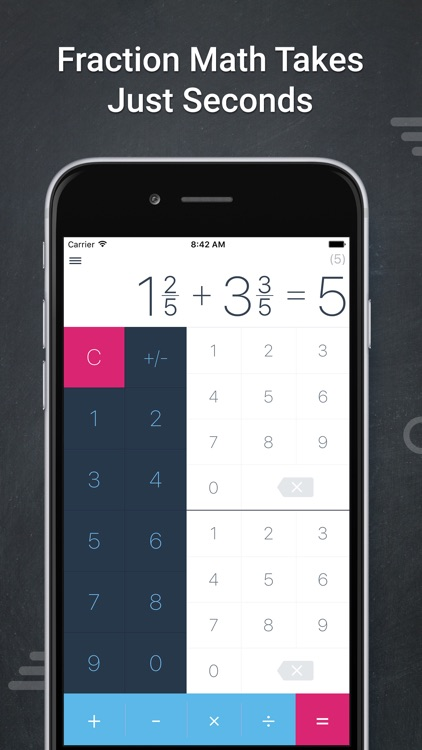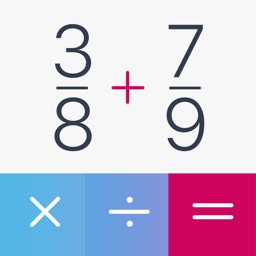### Fraction Calculator Pro Plus

Elena Polyanskaya

The best and easiest way to deal with everyday fraction problems. Fraction calculator will help you to check homework, work on a craft project, adjust recipe quantities. Attractive and Effective.

FEATURES:
- Checking fraction math takes just seconds!
- Add, subtract, multiply, divide fractions, quickly and clearly.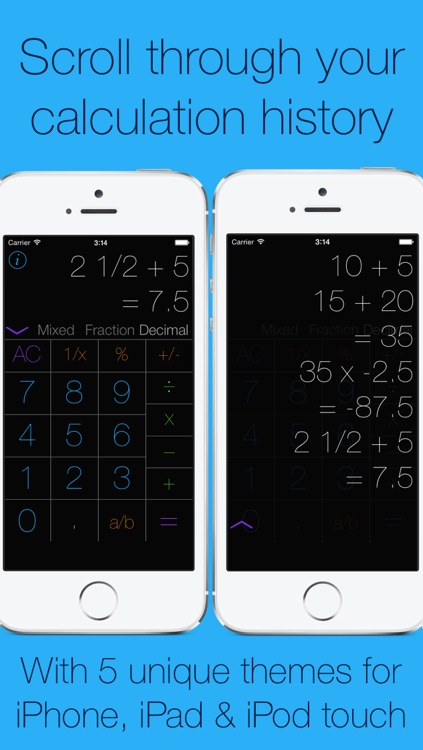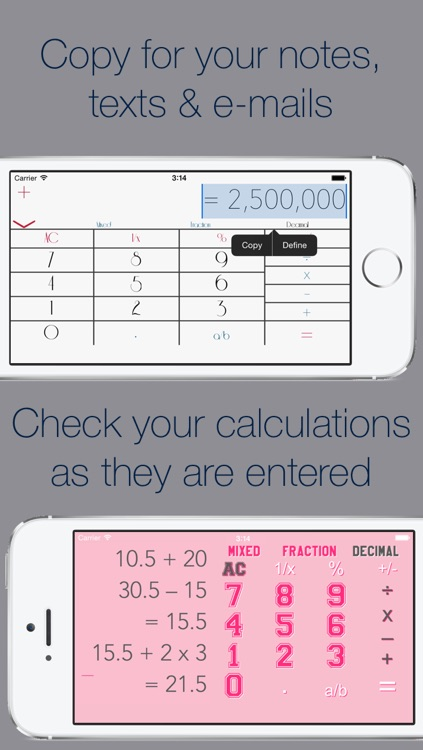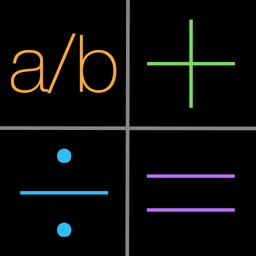### jCalc - Multi Calculator Free — with a history of your calculations plus a fraction calculator for iPhone, iPad and iPod touch

Jon Ericksen

Imagine a calculator that leaves all of your calculations visible and at your fingertips, never having to wonder if you typed in the correct numbers, and never again having to write down results as you start a new calculation.

Only 1.9 MB

jCalc Features:

• Drag down the display and scroll through...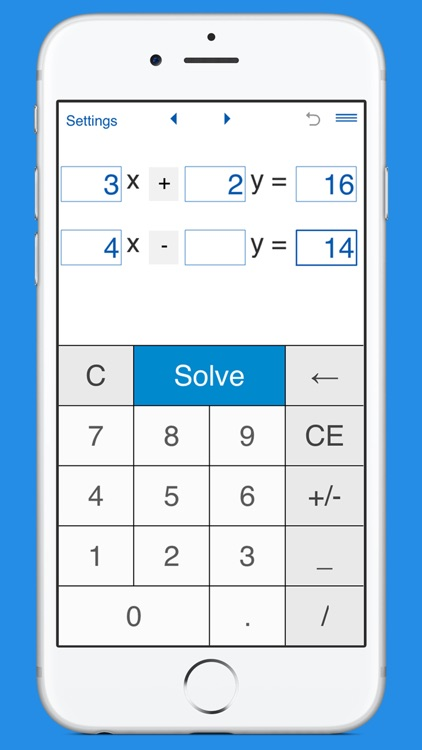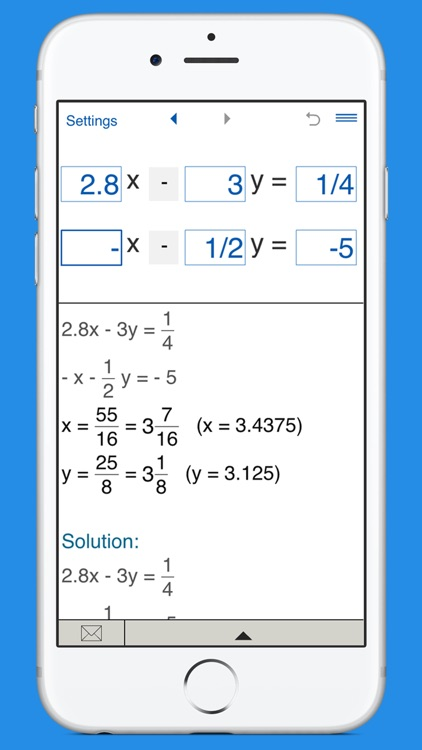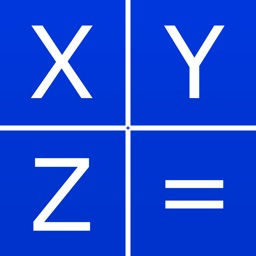### Systems of equations solver

Intemodino Group s.r.o.

The calculator solves systems of linear equations with two and three variables.

• System solver 2x2
Solves systems of two linear equations in two variables by substitution or using using Cramer's rule.

• System solver 3x3
Solves systems of three linear equations in three variables using Cramer's Rule.
To solve 3x3 systems of equations, you...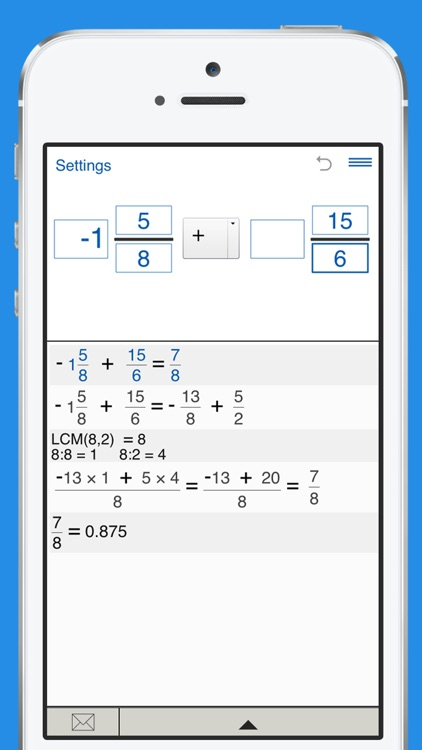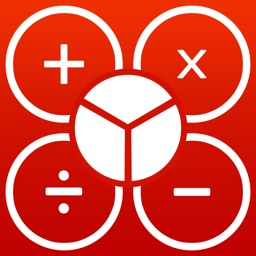### Fraction calculator with steps

Intemodino Group s.r.o.

Fraction calculator performs basic operations with fractions, mixed numbers and whole numbers.

Features:
• Fraction calculator for two and three fractions.
• Basic calculations: addition, subtraction, multiplication and division.
• Supports fractions, mixed numbers, and whole numbers.
•...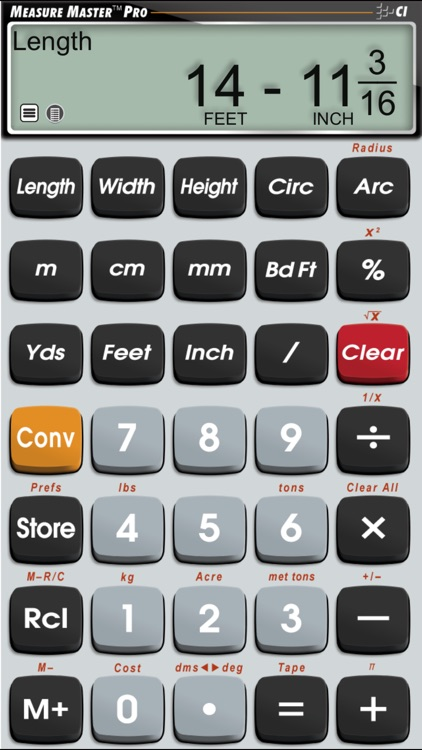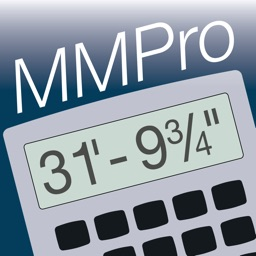### Measure Master Pro

Calculated Industries

Feet-Inch-Fraction and Metric Conversion Calculator by Calculated Industries®

The Measure Master Pro app from Calculated Industries gives you the power to solve U.S. and metric unit conversions on your iPhone or iPad. And while perhaps obvious, if you change from an iOS device to a non-iOS device, you WILL need to...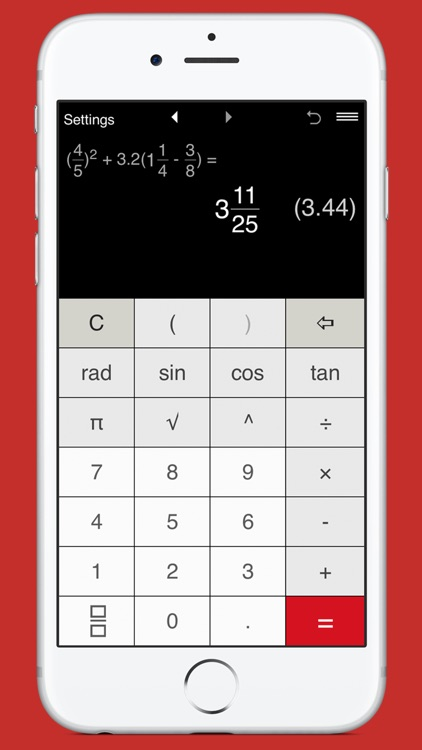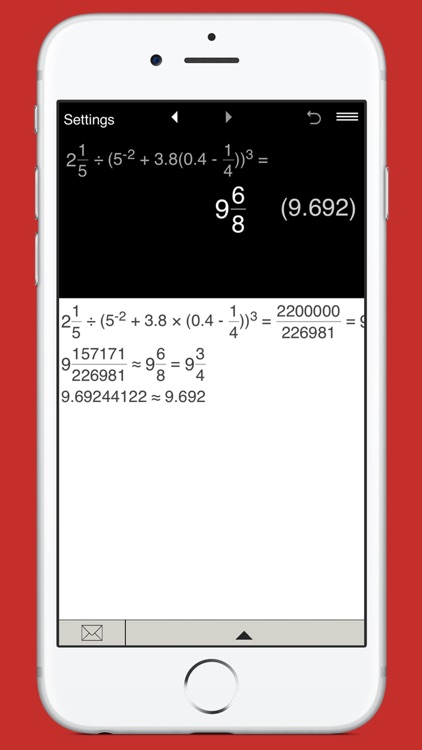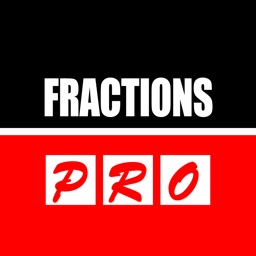### Fractions Pro

Intemodino Group s.r.o.

Get the all-in-one multifunctional calculator with fractions, designed to perform mathematical, engineering, scientific and technical calculations of any complexity. Powerful, easy to use and well designed advanced fraction calculator lets you perform calculations with proper and improper fractions, mixed numbers, decimals and integers. Thanks to a new improved design, added...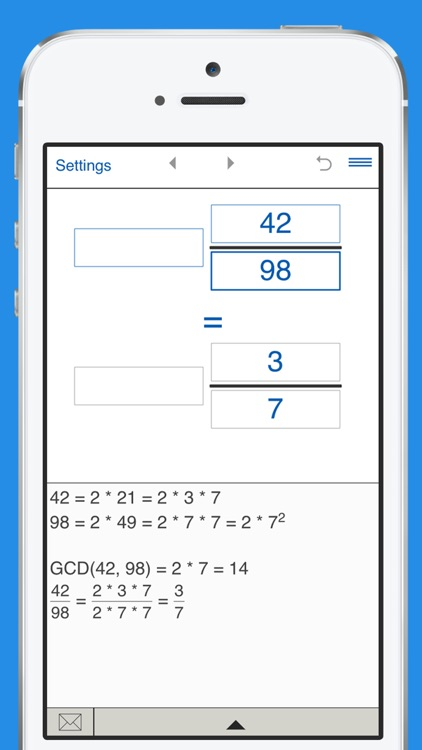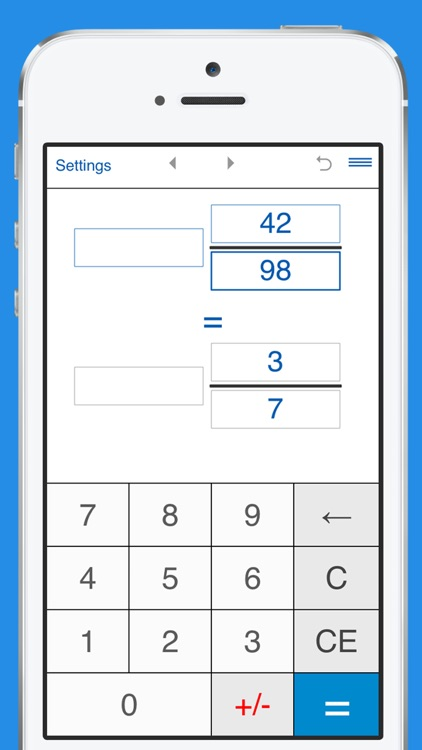### Simplify fractions calculator

Intemodino Group s.r.o.

A handy calculator that helps you reduce fractions and mixed numbers.

Features:
Reduces fractions to lowest terms.
Supports proper and improper fractions and mixed numbers.
Detailed results.
Finds the Greatest Common Factor (GCF) of the numerator and denominator.
Prime factorization of the numerator and denominator.
Keeps history list.
Sends results and history via email.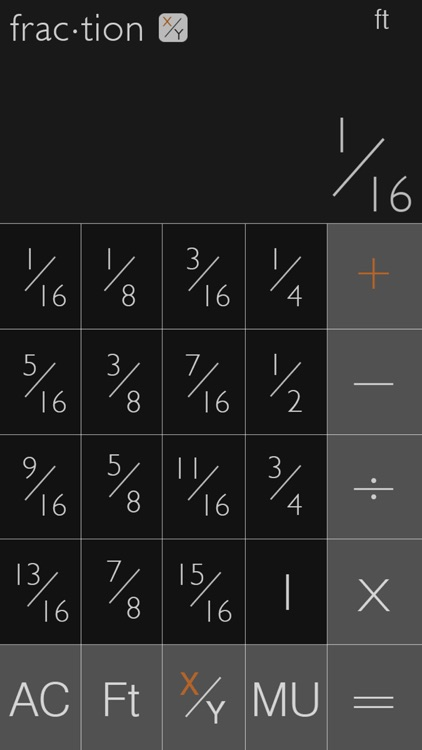### Frac·tion - fraction calculator

Clint Newsom

The calculator you've always needed, but never had. Fraction calculator adds, subtracts, multiplies, and divides fractions so you don't have to convert, but if you need to convert to decimals or just need a regular calculator, fraction does that too! Switch modes to convert from feet and inches to feet...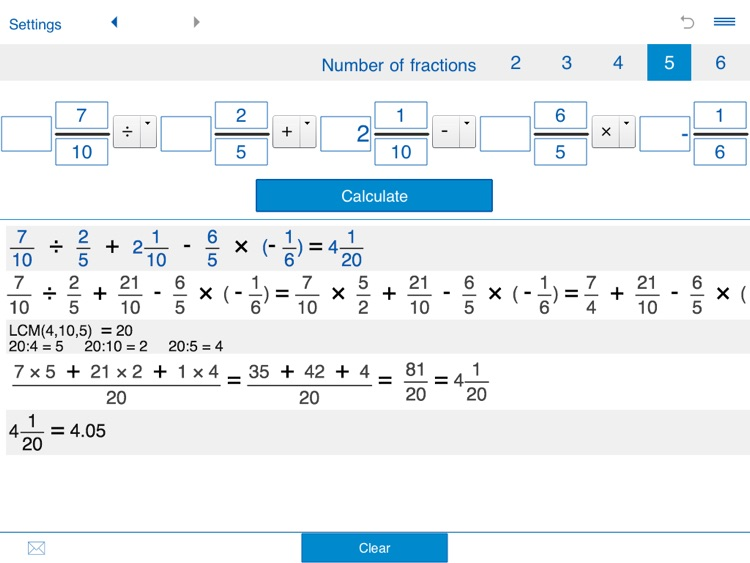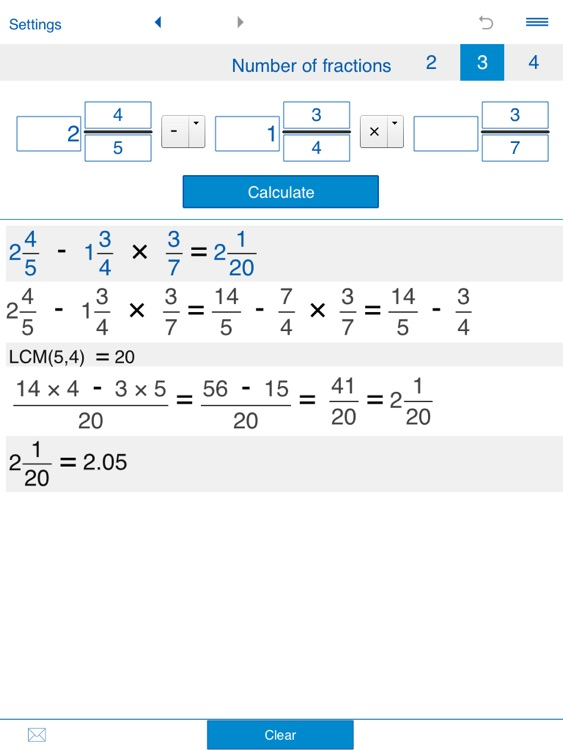### Fraction calculator XL

Intemodino Group s.r.o.

The best app for understanding, learning, teaching and calculating fractions!

Fraction calculator XL is an easy-to use fraction calculator with steps that enables you to quickly solve fraction problems and makes learning fractions much easier.

Fraction calculator...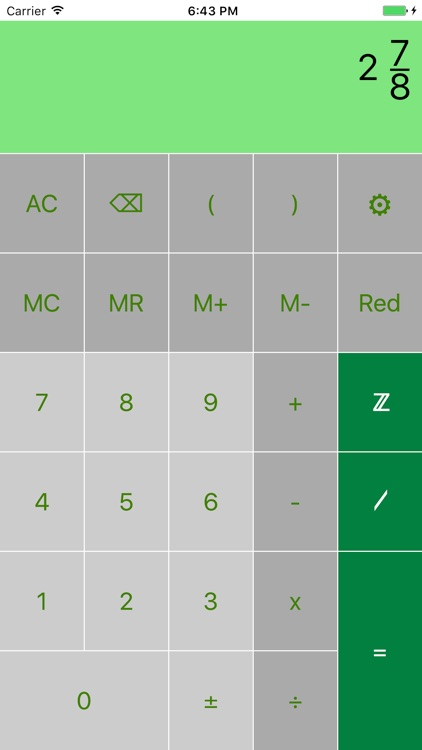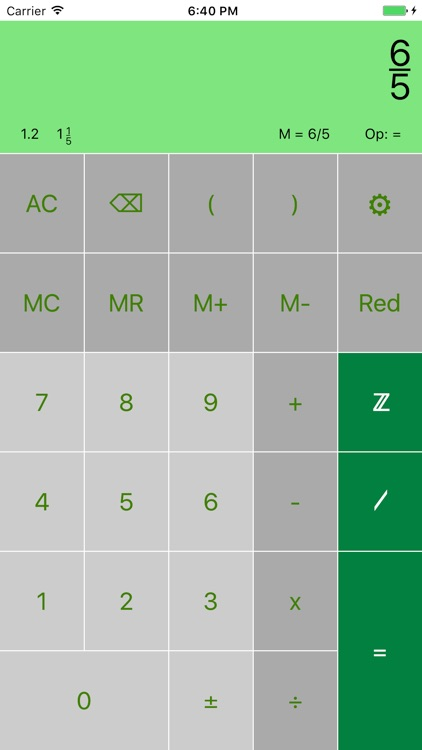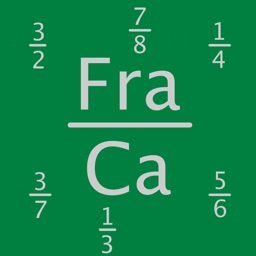### FraCa (fraction calculator)

Thomas Burow

FraCa is a calculator for fractions.

FraCa provides calculation using addition, subtraction, multiplication and division. In addition, the reducing of fractions is possible. Common fractions and mixed numbers can be use.

Additionally FraCa provides the calculation with parentheses and a simple memory function.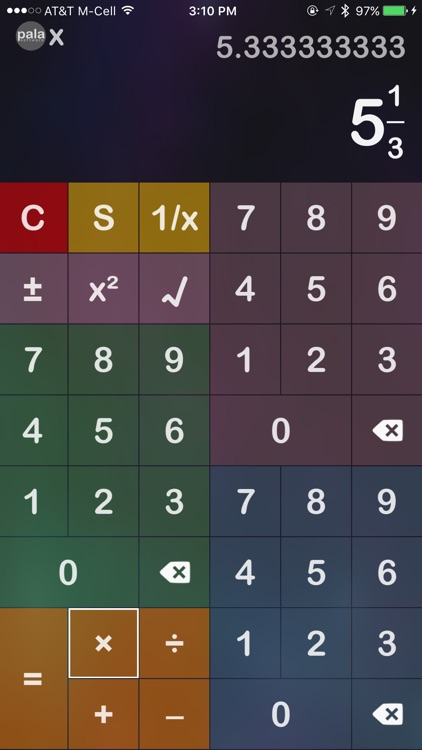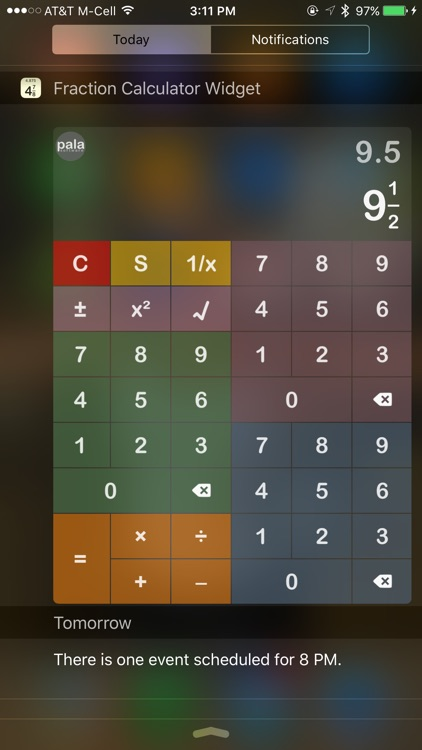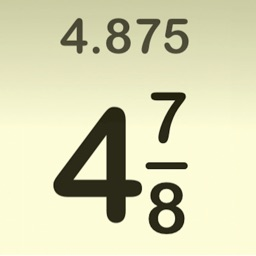### Fraction Calc by PalaSoftware

PalaSoftware Inc.

The Fraction Calculator feels and behaves exactly like a calculator with one exception, you're working with fractions. So now when homework (checking of course) calls for fractions, or your carpentry project needs you to add eighths and sixteenths dozens of times, or maybe you just want to be able to...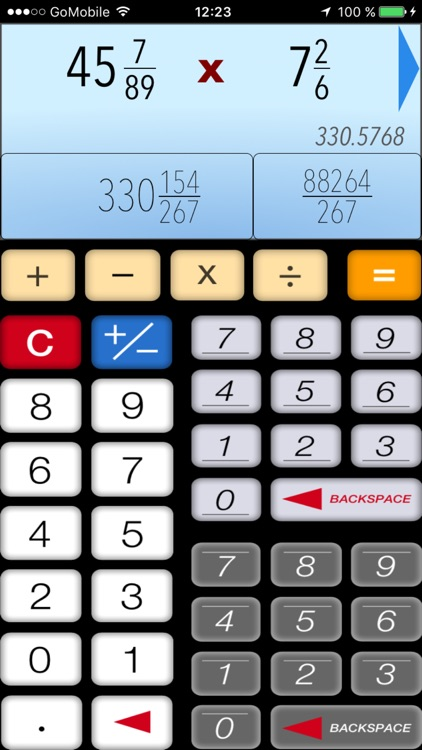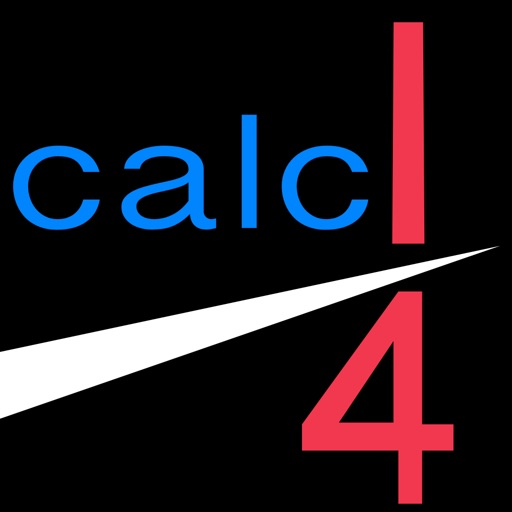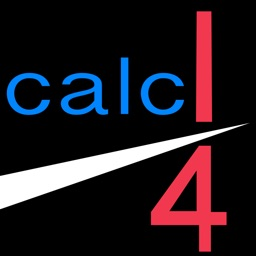### Calculator of Fractions

Gray Card Studio

Calculator of Fractions calculates simple and complex problems with fractions.
You can combine proper and improper fractions, mixed numbers and decimals in a single operation.
You can enter up to ten members (fractions, integers or decimals) in your calculation, each of them with up to 3 digits.
In case you...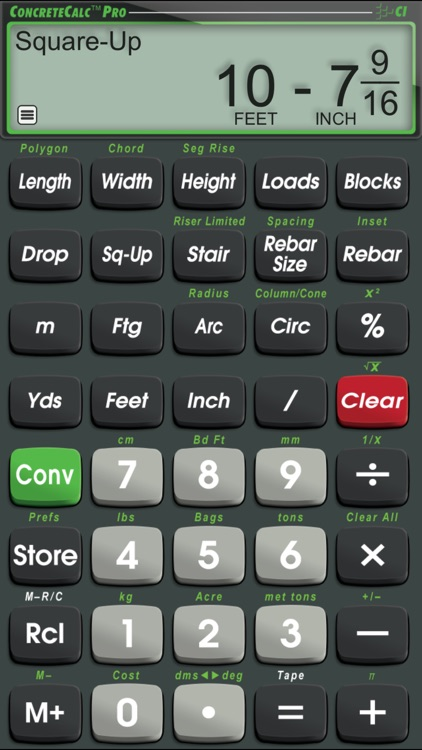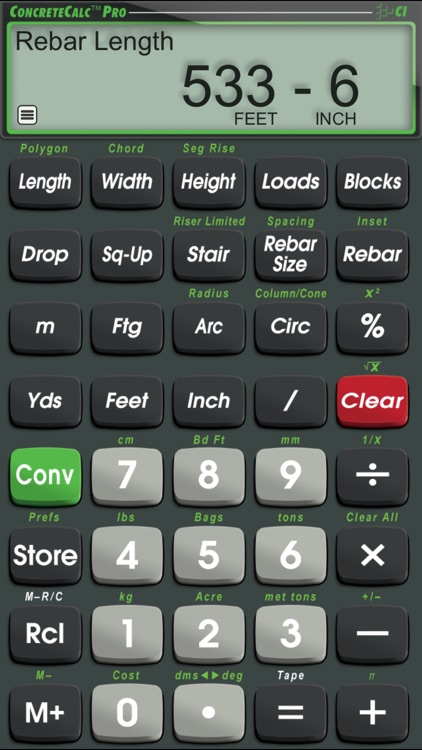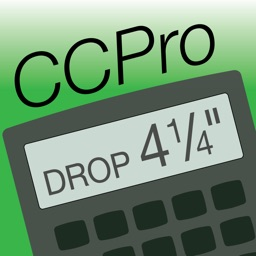### ConcreteCalc Pro

Calculated Industries

Advanced Concrete Construction-Math Calculator from Calculated Industries

The official ConcreteCalc Pro from Calculated Industries is now available for iPhone and iPad users. And while perhaps obvious, if you change from an iOS device to a non-iOS device, you WILL need to buy a new license as they are incompatible.

It helps concrete,...

## Other Apps

Could be good for special cases